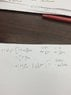# Checking if Momentum Operator is Hermitian - Integration

• MPKU

## Homework Statement

I'm checking to see if the momentum operator is Hermitian. Griffiths has the solution worked out, I'm just not following the integration by parts.

## Homework Equations

int(u dv) = uv - int(v du)

## The Attempt at a Solution

I've attached an image of my work.

It seems there should be an additional 'dx' with my 'v' term, but then the 'uv' portion would have a 'dx', which wouldn't make much sense to me.

•$$\frac{du}{dx} = \frac{df^*}{dx}$$
$$du = \frac{df^*}{dx} dx$$